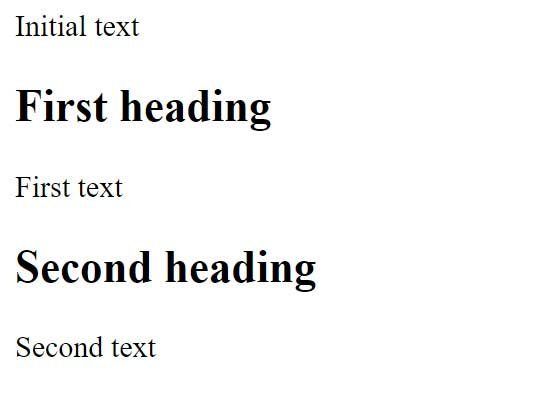# JavaScript code execution

When a browser receives a web page with html and JavaScript code, it interprets it. The result of interpretation in the form of various elements – buttons, input fields, text blocks, etc., we see in front of us in the browser. A web page is interpreted sequentially from top to bottom.

When a browser encounters an element with JavaScript on a web page <script>, the built-in JavaScript interpreter comes into play. And until he finishes his work, the interpretation of the web page does not go further.

Let’s consider a small example and for this we will change the index.html page from the previous topic as follows:

```<!DOCTYPE html>
<html lang="en">

<meta charset="UTF-8">
<meta http-equiv="X-UA-Compatible" content="IE=edge">
<meta name="viewport" content="width=device-width, initial-scale=1.0">
<title>JavaScript Tutorials</title>
<script>document.write("Initial text");</script>

<body>
<script>document.write("First text");</script>
<script>document.write("Second text");</script>
</body>

</html>
```

There are three JavaScript code inserts – one in the section <head>and one after each heading.

Let’s open the web page in the browser and we will see that the browser sequentially executes the web page code:Here we see that the JavaScript code from the head section is executed first, which displays some text on the web page:

` document.write("Initial text"); `

Next, the first standard html element is displayed <h2>:

` <h2>First text</h2> `

After that, the second insertion of the JavaScript code is performed:

` document.write("First text"); `

Then the second html element is rendered <h2>:

`  <h2>Second title </h2> `

And at the end, the last insertion of the JavaScript code is performed:

` document.write("Second text"); `

The browser will then finish interpreting the web page and the web page will be fully loaded. This point is very important because it can affect performance. Therefore, it is not uncommon for JavaScript code insertions to come before the closing </body> tag when the main part of the web page has already loaded in the browser.

JavaScript Syntax Basics
Before moving on to a detailed study of the basics of the JavaScript programming language, let’s look at some basic points of its syntax.

The JavaScript code is made up of instructions. Each instruction represents some action. And to separate instructions from each other in JavaScript, a semicolon is placed after the instruction:

```<!DOCTYPE html>
<html lang="en">

<meta charset="UTF-8">
<meta http-equiv="X-UA-Compatible" content="IE=edge">
<meta name="viewport" content="width=device-width, initial-scale=1.0">
<title>JavaScript Tutorials</title>
<script>document.write("Initial text");</script>

<body>

<script>document.write("2 + 5 =  "); var sum = 2 + 5; document.write(sum);</script>
</body>

</html>
```

There are three instructions defined in the JavaScript code here:

• Displays the text “2 + 5 = ” on the page
` document.write("2 + 5 =  ")  `
• Using the var statement , defines the variable sum , which is the sum of 2 + 5
` var sum = 2 + 5;  `
• Displays the value of the variable sum (that is, the sum of 2 + 5) on the page
` document.write(sum); `

However, modern browsers may well distinguish between individual instructions if they are simply on separate lines without a semicolon:

```<!DOCTYPE html>
<html lang="en">

<meta charset="UTF-8">
<meta http-equiv="X-UA-Compatible" content="IE=edge">
<meta name="viewport" content="width=device-width, initial-scale=1.0">
<title>JavaScript Tutorials</title>
<script>document.write("Initial text");</script>

<body>

<script>
document.write("2 + 5 =  "); /* Comment Goes here*/
var sum = 2 + 5; /* Comment Goes here*/
document.write(sum); /* Comment Goes here*/
</script>
</body>

</html>
```

But in order to improve the readability of the code and reduce the number of possible errors, it is recommended to define each JavaScript instruction on a separate line and end it with a semicolon, as in the first option.

Comments can be used in JavaScript code. Comments are not processed by the JavaScript interpreter and are not taken into account in any way in the program. They are for code orientation, to indicate what a particular code does.

```<!DOCTYPE html>
<html lang="en">

<meta charset="UTF-8">
<meta http-equiv="X-UA-Compatible" content="IE=edge">
<meta name="viewport" content="width=device-width, initial-scale=1.0">
<title>JavaScript Tutorials</title>
<script>document.write("Initial text");</script>

<body>

<script>
document.write("2 + 5 =  "); // single line Comment Goes here
var sum = 2 + 5; // single line Comment Goes here
document.write(sum); // single line Comment Goes here
</script>
</body>

</html>
```

Comments can be single-line, for which a double slash is used:

For example:

```<!DOCTYPE html>
<html lang="en">

<meta charset="UTF-8">
<meta http-equiv="X-UA-Compatible" content="IE=edge">
<meta name="viewport" content="width=device-width, initial-scale=1.0">
<title>JavaScript Tutorials</title>
<script>document.write("Initial text");</script>

<body>

<script>
document.write("2 + 5 =  ");
/* An example of an arithmetic operation and variable
definitions in JavaScript code */
var sum = 2 + 5; // single line Comment Goes here
document.write(sum); // single line Comment Goes here
</script>
</body>

</html>
```